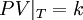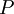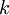# Boyle's law$PV\vert_T=k$
where$P$ is the pressure,$V$ is the volume and$k$ is a constant. This holds true for an ideal gas.